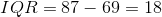# AP Statistics : How to interpret stemplots

## Example Questions

### Example Question #1 : How To Interpret Stemplots

Find the range of the data in the stem-and-leaf plot.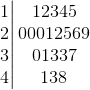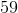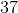Explanation:

To find the range, subtract the minimum value from the maximum value

minimum:maximum:So,

maximum - minimum =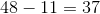### Example Question #2 : How To Interpret Stemplots

Find the median of the stem and leaf plot.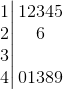Explanation:

The median is the middle value of the set in increasing order.

In this set of 11 entries, the median is the 6th entry of the set in increasing order, or 26.

### Example Question #3 : How To Interpret Stemplots

How many entries are in this stem and leaf plot?Explanation:

The number of entries is the number of digits on the right hand side of the column.

Since there are 21 total digits, there are 21 total entris that make up the stem and leaf plot.

### Example Question #4 : How To Interpret Stemplots

What is the interquartile range of the following data set?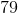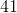Explanation:

This stemplot is read as follows: the stem is the tens digit and each digit in the "leaves" section is a ones digit. Put them together to have a data point.

53, 65, 68, 69, 70, 72, 72, 79, 83, 84, 85, 87, 89, 90, 94

In the particular case there are 15 data points therefore the median is 79. Thus the first quartile is 69 and the third quartile is 87.

Finding the interquartile range is subtracting the first quartile from the 3rd quartile.